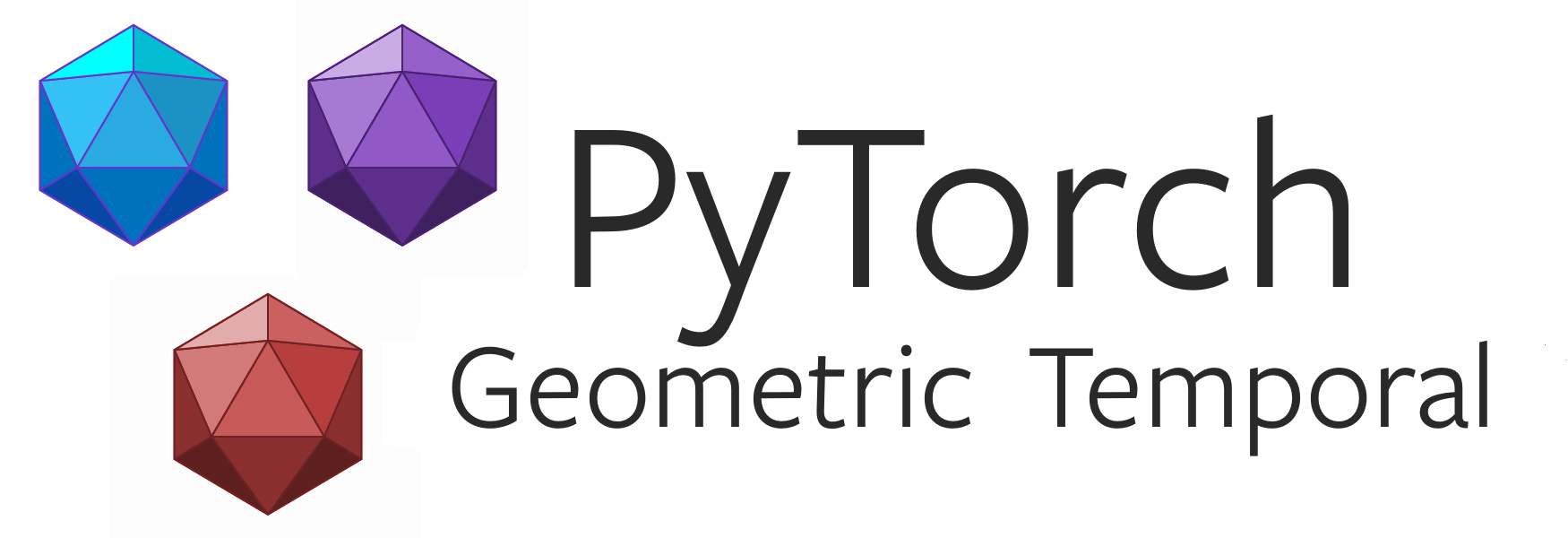PyTorch Geometric Temporal is a temporal (dynamic) extension library for PyTorch Geometric.

The library consists of various dynamic and temporal geometric deep learning, embedding, and spatio-temporal regression methods from a variety of published research papers. Moreover, it comes with an easy-to-use dataset loader, train-test splitter and temporal snaphot iterator for dynamic and temporal graphs. The framework naturally provides GPU support. It also comes with a number of benchmark datasets from the epidemological forecasting, sharing economy, energy production and web traffic management domains. Finally, you can also create your own datasets.

The package interfaces well with Pytorch Lightning which allows training on CPUs, single and multiple GPUs out-of-the-box. Take a look at this introductory example of using PyTorch Geometric Temporal with Pytorch Lighning.

We also provide detailed examples for each of the recurrent models.

Case Study Tutorials

We provide in-depth case study tutorials in the Documentation, each covers an aspect of PyTorch Geometric Temporal’s functionality.

Incremental TrainingEpidemiological Forecasting Case Study

Cumulative TrainingWeb Traffic Management Case Study

Citing

If you find PyTorch Geometric Temporal and the new datasets useful in your research, please consider adding the following citation:

``````@inproceedings{rozemberczki2021pytorch,
author = {Benedek Rozemberczki and Paul Scherer and Yixuan He and George Panagopoulos and Alexander Riedel and Maria Astefanoaei and Oliver Kiss and Ferenc Beres and Guzman Lopez and Nicolas Collignon and Rik Sarkar},
title = {{PyTorch Geometric Temporal: Spatiotemporal Signal Processing with Neural Machine Learning Models}},
year = {2021},
booktitle={Proceedings of the 30th ACM International Conference on Information and Knowledge Management},
}
``````

A simple example

PyTorch Geometric Temporal makes implementing Dynamic and Temporal Graph Neural Networks quite easy - see the accompanying tutorial. For example, this is all it takes to implement a recurrent graph convolutional network with two consecutive graph convolutional GRU cells and a linear layer:

``````import torch
import torch.nn.functional as F
from torch_geometric_temporal.nn.recurrent import GConvGRU

class RecurrentGCN(torch.nn.Module):

def __init__(self, node_features, num_classes):
super(RecurrentGCN, self).__init__()
self.recurrent_1 = GConvGRU(node_features, 32, 5)
self.recurrent_2 = GConvGRU(32, 16, 5)
self.linear = torch.nn.Linear(16, num_classes)

def forward(self, x, edge_index, edge_weight):
x = self.recurrent_1(x, edge_index, edge_weight)
x = F.relu(x)
x = F.dropout(x, training=self.training)
x = self.recurrent_2(x, edge_index, edge_weight)
x = F.relu(x)
x = F.dropout(x, training=self.training)
x = self.linear(x)
return F.log_softmax(x, dim=1)
``````

Methods Included

In detail, the following temporal graph neural networks were implemented.

Recurrent Graph Convolutions

Expand to see all implemented recurrent graph convolutional layers...

Attention Aggregated Temporal Graph Convolutions

Expand to see all implemented attention aggregated graph convolutional layers...

Auxiliary Graph Convolutions

Expand to see all auxiliary graph convolution layers...

Head over to our documentation to find out more about installation, creation of datasets and a full list of implemented methods and available datasets. For a quick start, check out the examples in the `examples/` directory.

If you notice anything unexpected, please open an issue. If you are missing a specific method, feel free to open a feature request.

Installation

Binaries are provided for Python version <= 3.9.

PyTorch 1.9.0

To install the binaries for PyTorch 1.9.0, simply run

``````pip install torch-scatter -f https://pytorch-geometric.com/whl/torch-1.9.0+\${CUDA}.html
pip install torch-sparse -f https://pytorch-geometric.com/whl/torch-1.9.0+\${CUDA}.html
pip install torch-cluster -f https://pytorch-geometric.com/whl/torch-1.9.0+\${CUDA}.html
pip install torch-spline-conv -f https://pytorch-geometric.com/whl/torch-1.9.0+\${CUDA}.html
pip install torch-geometric
pip install torch-geometric-temporal
``````

where `\${CUDA}` should be replaced by either `cpu`, `cu102`, or `cu111` depending on your PyTorch installation.

`cpu` `cu102` `cu111`
Linux
Windows
macOS

PyTorch 1.8.0

To install the binaries for PyTorch 1.8.0, simply run

``````\$ pip install torch-scatter -f https://pytorch-geometric.com/whl/torch-1.8.0+\${CUDA}.html
\$ pip install torch-sparse -f https://pytorch-geometric.com/whl/torch-1.8.0+\${CUDA}.html
\$ pip install torch-cluster -f https://pytorch-geometric.com/whl/torch-1.8.0+\${CUDA}.html
\$ pip install torch-spline-conv -f https://pytorch-geometric.com/whl/torch-1.8.0+\${CUDA}.html
\$ pip install torch-geometric
\$ pip install torch-geometric-temporal
``````

where `\${CUDA}` should be replaced by either `cpu`, `cu101`, `cu102`, or `cu111` depending on your PyTorch installation.

`cpu` `cu101` `cu102` `cu111`
Linux
Windows
macOS

PyTorch 1.7.0

To install the binaries for PyTorch 1.7.0, simply run

``````\$ pip install torch-scatter==latest+\${CUDA} -f https://pytorch-geometric.com/whl/torch-1.7.0.html
\$ pip install torch-sparse==latest+\${CUDA} -f https://pytorch-geometric.com/whl/torch-1.7.0.html
\$ pip install torch-cluster==latest+\${CUDA} -f https://pytorch-geometric.com/whl/torch-1.7.0.html
\$ pip install torch-spline-conv==latest+\${CUDA} -f https://pytorch-geometric.com/whl/torch-1.7.0.html
\$ pip install torch-geometric
\$ pip install torch-geometric-temporal
``````

where `\${CUDA}` should be replaced by either `cpu`, `cu92`, `cu101`, `cu102` or `cu110` depending on your PyTorch installation.

`cpu` `cu92` `cu101` `cu102` `cu110`
Linux
Windows
macOS

PyTorch 1.6.0

To install the binaries for PyTorch 1.6.0, simply run

``````\$ pip install torch-scatter==latest+\${CUDA} -f https://pytorch-geometric.com/whl/torch-1.6.0.html
\$ pip install torch-sparse==latest+\${CUDA} -f https://pytorch-geometric.com/whl/torch-1.6.0.html
\$ pip install torch-cluster==latest+\${CUDA} -f https://pytorch-geometric.com/whl/torch-1.6.0.html
\$ pip install torch-spline-conv==latest+\${CUDA} -f https://pytorch-geometric.com/whl/torch-1.6.0.html
\$ pip install torch-geometric
\$ pip install torch-geometric-temporal
``````

where `\${CUDA}` should be replaced by either `cpu`, `cu92`, `cu101` or `cu102` depending on your PyTorch installation.

`cpu` `cu92` `cu101` `cu102`
Linux
Windows
macOS

Running tests

``````\$ python setup.py test
``````

Get A Weekly Email With Trending Projects For These Topics
No Spam. Unsubscribe easily at any time.
Python (1,141,503
Deep Learning (22,844
Pytorch (11,070
Graph Neural Networks (540
Spatial Analysis (226
Graph Convolutional Networks (208
Gcn (164
Spatial Data (155
Gnn (140
Related Projects